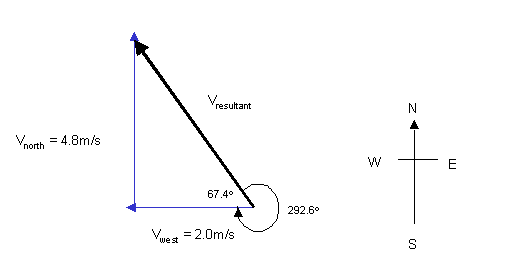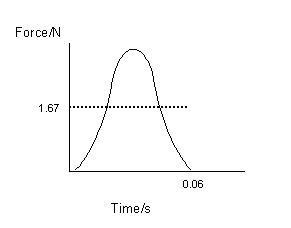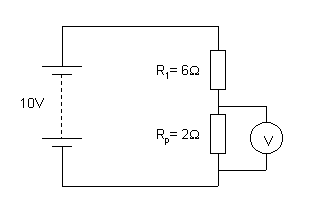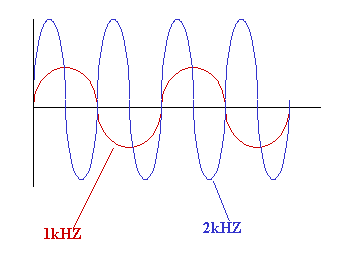## 1992 Higher Physics Paper I Solutions

```1. E		11. E			21. B
2. A		12. B			22. C
3. C		13. D			23. C
4. C		14. D			24. B
5. E		15. A			25. A
6. E		16. B			26. B
7. B		17. B			27. D
8. C		18. A			28. C
9. E		19. B			29. C
10.E		20. E			30. C

31. Draw a scaled diagram or use trigonometry/Pythagoras' theorem to solve.Vresultant2 = Vnorth2+Vwest2
Vresultant2 = 4.82+2.02
Vresultant2 = 23.04+4.0
Vresultant2 = 27.04
Vresultant = 5.2m/s

tanq = Vnorth/Vwest
tanq = 4.8/2
tanq = 2.4
q = tan-12.4
q = 67.4o

Vresultant = 5.2m/s at a bearing of 292.6o

32.a.	Favg = DP/t
Favg = m(v-u)/t

m = 0.05kg
u = 0m/s
v = 2.0m/s
t = 0.060s

Favg = 0.05(2.0-0)/0.06
Favg = 1.67N

b.33.a.	Vmeter = [R1/(R1+R2)]xVsupply
Vmeter = [6/(6+6)]x10
Vmeter = 5V

This answer is obvious because the voltage splits up evenly
across the 6W resistors.

b.	Calculate the resistance of the 3W and 6W parallel network.

1/Rp = 1/R1 + 1/R2
1/Rp = 1/6 +1/3
1/Rp = 3/6
Rp = 6/3 = 2W

The circuit can therefore be reduced to that below.Where the 2W resistor represents the parallel network.

The voltmeter reading can now be calculated.

Vmeter = [Rp/(R1+Rp)]xVsupply
Vmeter = [2/(6+2)]x10
Vmeter = 2.5V

This problem can also be solved using ohm's law.

34.	Each half of the loop has a resistance of 15W. These half loops
are like two resistors of equal value in parallel. Using this
analysis allows Rp to be calculated.

Rp = R/N
Rp = 15/2
Rp = 7.5W

35.a.	This is an example of a nuclear fission reaction.

b.	The total mass of the reactants is greater than the mass of
the products. This "missing mass" also called the mass defect
is converted into energy.

The energy released can be calculated using E = mc2, where m
is the missing mass value in kilograms.

36.To increase the current in the circuit, as the supply frequency is
increased, the voltage across the resistor must increase.

37.a.	E4 to E0 will produce the highest energy/frequency radiation.

b.	DE = E4 - E0
DE = hf

f = DE/h
f = (E4 - E0)/h
f = [-0.864x10-19-(-21.76x10-19)]/6.63x10-34
f = 20.896x10-19/6.63x10-34
f = 3.15x1015Hz

END OF QUESTION PAPER